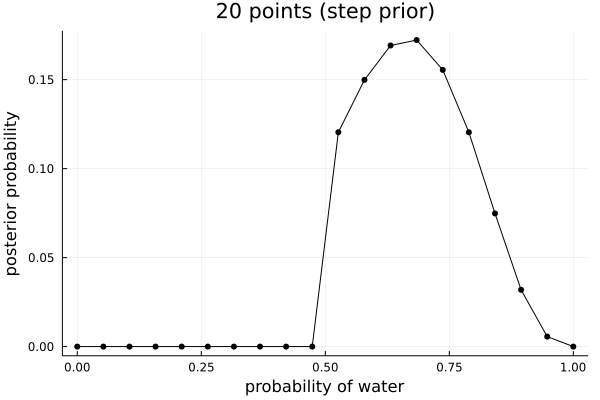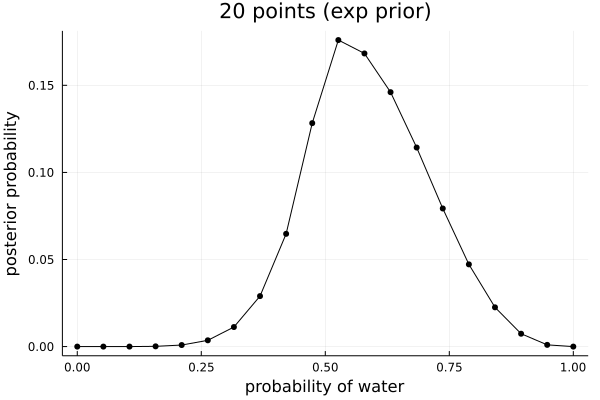## Statistical Rethinking #

Statistical Rethinking is a textbook by Richard McElreath which builds up the foundations of statistics through thinking about models instead of test, taking an entirely Bayesian approach. These are some notes I made and thoughts I had while reading the book.

### a.external { background: url('/images/external.png') no-repeat 100% 0; background-size: 14px 14px; padding-right: 16px; } Julia code #

I am trying to reproduce all the code in the book in Julia as an excuse to learn the language.

#### 2. Small worlds and large worlds #

• 2.1 The Garden of Forking Data

• 2.1.1-2 Counting possibilities and combining other information

Gives a nice “garden of forked data” example with a simple blue/white ball setup. Similar to the approach by Gelman at al (Bayesian statistics).

• 2.1.3 From counts to probability

In Julia we can use the norm function from the LinearAlgebra package in the standard library to normalise the frequency vector in a vector of “plausibilities”.

using LinearAlgebra
x = [0, 3, 8, 9, 0];
print(x / norm(x, 1))

5-element Vector{Int64}:
0
3
8
9
0
[0.0, 0.15, 0.4, 0.45, 0.0]

• 2.2 Building a model

• 2.2.1 A data story
• 2.2.2 Bayesian updating
• 2.2.3 Evaluate
• 2.3 Components of the model

• 2.3.1 Variables
• 2.3.2 Definitions

In Julia let’s build the binomial density function ourselves by implementing the formula at the bottom of page 33,

$P(W, L\mid p) = \frac{(W+L)!}{W!L!}p^W (1-p)^W$

using Distributions

function dbinom(n::Integer, size::Integer, prob::Real)
binomial(size, n) * prob^n * (1.0-prob)^(size-n)
end;

dbinom( 6, 9, 0.5 )

dbinom (generic function with 1 method)
0.1640625

• 2.3.3 A model is born
• 2.4 Making the model go

• 2.4.1 Bayes' Theorem
• 2.4.2 Motors
• 2.4.3 Grid approximation

Here we will implement the simple Binomial grid approximation example in Julia.

using Plots
using Distributions

function dbinom(n::Integer, size::Integer, prob::Real)
binomial(size, n) * prob^n * (1.0-prob)^(size-n)
end;

p_grid = collect(0:1/19:1)
prior = fill(1, 20)
likelihood = dbinom.( Ref(6), Ref(9), p_grid)
unstandardised_posterior = likelihood .* prior
posterior = unstandardised_posterior / sum(unstandardised_posterior)
f = plot(p_grid,
posterior,
markershape = :circle,
xlabel="probability of water",
ylabel="posterior probability",
title="20 points",
color=:black,
legend=false)
savefig(f, "~/roam/img/rethinking_stats_2_4_3.png")


And with the other two priors suggested:

step_prior = (x -> if x > .5 1 else 0 end).(p_grid)
exp_prior = (x -> exp( -5 * abs( x - .5 ) )).(p_grid)